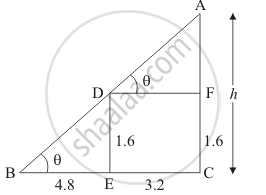# A 1.6 M Tall Girl Stands at a Distance of 3.2 M from a Lamp-post and Casts a Shadow of 4.8 M on the Ground. Find the Height of the Lamp-post by Using (I) Trigonometric Ratios (Ii) Property of Similar Triangles. - Mathematics

A 1.6 m tall girl stands at a distance of 3.2 m from a lamp-post and casts a shadow of 4.8 m on the ground. Find the height of the lamp-post by using (i) trigonometric ratios (ii) property of similar triangles.

#### Solution

Let AC be the lamp post of height h

We assume that ED 1.6 m, BE = 4.8 m and EC = 3.2 m

We have to find the height of the lamp post

Now we have to find the height of lamp post using similar triangles.Since triangle BDE and triangle ABC are similar.

(AC)/(BC) = (ED)/(BE)

h/(4.8 + 3.2) = 1.6/(BE)

h =  8/3

Again, we have to find height of lamp post using trigonometric ratios.

=> tan theta = 1.6/4.8

=> tan theta  = 1/3

Again in  ΔABC

=> tan theta = h/(4.8 + 3.2)

=> 1/2  = h/8

h = 8/3

Hence the height of lamp post is 8/3 m

Concept: Heights and Distances
Is there an error in this question or solution?

#### APPEARS IN

RD Sharma Class 10 Maths
Chapter 12 Trigonometry
Exercise 12.1 | Q 21 | Page 31